# Surface area of a cube or a rectangular prism Online Quiz

#### Surface Areas and Volumes

117 Lectures 6.5 hours

#### Class 10th - Surface Areas and Volumes

20 Lectures 1 hours

#### Class 10th - Surface Areas and Volumes (Hindi)

10 Lectures 47 mins

Following quiz provides Multiple Choice Questions (MCQs) related to Surface area of a cube or a rectangular prism. You will have to read all the given answers and click over the correct answer. If you are not sure about the answer then you can check the answer using Show Answer button. You can use Next Quiz button to check new set of questions in the quiz.Q 1 - Find the surface area of the given rectangular prism in square cm.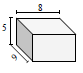### Explanation

Step 1:

Surface area of a rectangular prism = 2(lw + wh + 1h); l = 8 cm; w = 5 cm; h = 9 cm

Step 2:

Surface area of given prism = 2(8×5 + 8×9 + 9×5)

= 2(40 + 72 + 45)

= 314 square cm

Q 2 - Find the surface area of the given rectangular prism in square cm.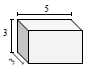### Explanation

Step 1:

Surface area of a rectangular prism = 2(lw + wh + 1h); l = 5 cm; w = 3 cm; h = 3 cm

Step 2:

Surface area of given prism = 2(5×3 + 5×3 + 3×3)

= 2(15 + 15 + 9)

= 78 square cm

Q 3 - Find the surface area of the given rectangular prism in square cm.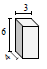### Explanation

Step 1:

Surface area of a rectangular prism = 2(lw + wh + 1h); l = 3 cm; w = 6 cm; h = 4 cm

Step 2:

Surface area of given prism = 2(3×6 + 3×4 + 6×4)

= 2(18 + 12 + 24)

= 108 square cm

Q 4 - Find the surface area of the given rectangular prism in square cm.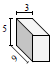### Explanation

Step 1:

Surface area of a rectangular prism = 2(lw + wh + 1h); l = 3 cm; w = 5 cm; h = 9 cm

Step 2:

Surface area of given prism = 2(3×5 + 3×9 + 9×5)

= 2(15 + 27 + 45)

= 174 square cm

Q 5 - Find the surface area of the given rectangular prism in square cm.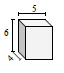### Explanation

Step 1:

Surface area of a rectangular prism = 2(lw + wh + 1h); l = 5 cm; w = 6 cm; h = 4 cm

Step 2:

Surface area of given prism = 2(5×6 + 5×4 + 6×4)

= 2(30 + 20 + 24)

= 148 square cm

Q 6 - Find the surface area of the given rectangular prism in square cm.### Explanation

Step 1:

Surface area of a rectangular prism = 2(lw + wh + 1h); l = 6 cm; w =9 cm; h = 6 cm

Step 2:

Surface area of given prism = 2(6×9 + 6×6 + 9×6)

= 2(54 + 36 + 54)

= 288 square cm

Q 7 - Find the surface area of the given rectangular prism in square cm.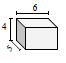### Explanation

Step 1:

Surface area of a rectangular prism = 2(lw + wh + 1h); l = 6 cm; w = 4 cm; h = 5 cm

Step 2:

Surface area of given prism = 2(6×4 + 6×5 + 4×5)

= 2(24 + 30 + 20)

= 148 square cm

Q 8 - Find the surface area of the given rectangular prism in square cm.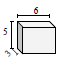### Explanation

Step 1:

Surface area of a rectangular prism = 2(lw + wh + 1h); l = 6 cm; w = 5 cm; h = 3 cm

Step 2:

Surface area of given prism = 2(6×5 + 6×3 + 5×3)

= 2(30 + 18 + 15)

= 126 square cm

Q 9 - Find the surface area of the given rectangular prism in square cm.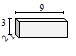### Explanation

Step 1:

Surface area of a rectangular prism = 2(lw + wh + 1h); l = 9 cm; w = 3 cm; h = 2 cm

Step 2:

Surface area of given prism = 2(9×3 + 9×2 + 3×2)

= 2(27 + 18 + 6)

= 102 square cm

Q 10 - Find the surface area of the given rectangular prism in square cm.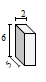### Explanation

Step 1:

Surface area of a rectangular prism = 2(lw + wh + 1h); l = 2 cm; w = 6 cm; h = 5 cm

Step 2:

Surface area of given prism = 2(2×6 + 2×5 + 6×5)

= 2(12 + 10 + 30)

= 104 square cm

surface_area_of_cube_or_rectangular_prism.htm